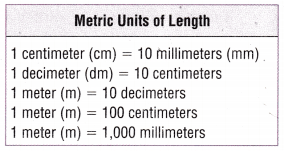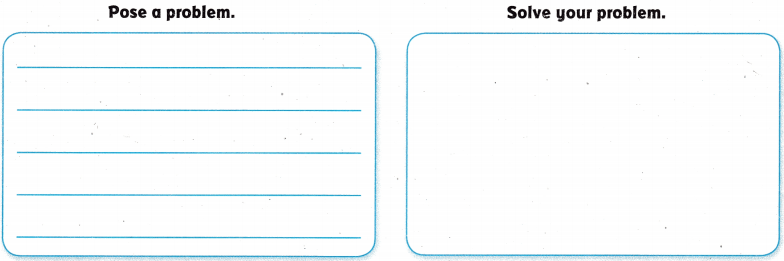# Texas Go Math Grade 4 Lesson 15.6 Answer Key Metric Units of Length

Refer to our Texas Go Math Grade 4 Answer Key Pdf to score good marks in the exams. Test yourself by practicing the problems from Texas Go Math Grade 4 Lesson 15.6 Answer Key Metric Units of Length.

## Texas Go Math Grade 4 Lesson 15.6 Answer Key Metric Units of Length

Essential Question

How can you convert metric units of length?
Answer: To convert a smaller unit to a larger unit (eg to ), divide it by the number of smaller units which are needed to make. To convert from a larger unit to a smaller one, multiply. To convert from a smaller unit to a larger one, divide.

InvestigateMaterials

• ruler (meter)
• scissors
• tape

Meters (in), decimeters (dm), centimeters (cm), and millimeters (mm) aie all metric units of length.

Build a meterstick to show how these units are related.

A. Cut out the meterstick strips.
1 meter = 10 decimeter

B. Place the strips end-to-end to build 1 meter. Tape the strips together.
10 decimeter strips to make 1 meter.

C. Look at your meter strip. What patterns do you notice about the sizes of the units?
1 meter is ________ times as long as 1 decimeter.
1 decimeter is ________ times as long as 1 centimeter.
1 centimeter is ________ times as long as 1 millimeter.
1 meter is 10 times as long as 1 decimeter.
1 decimeter is 10  times as long as 1 centimeter.
1 centimeter is 10 times as long as 1 millimeter.

Describe the pattern you see.
Each is multiplied with 10

Math Idea: If you lined up 1,000 metersticks end-to-end, the length of the metersticks would be 1 kilometer.

H.O.T. Apply What operation could you use to find how many centimeters are in 3 meters? Explain.
1 meter = 100 centimeters
3 x100 = 300
Multiplication operation is used.

Make Connections

You can use different metric units to describe the same metric length. For example, you can measure the length of a book as 3 decimeters or as 30 centimeters. Since the metric system is based on the number 10, decimals or fractions can be used to describe metric lengths as equivalent units.

Think of 1 meter as one whole. Use your meterstick to write equivalent units as fractions and decimals.

1 meter = 10 decimeters
Each decimeter is
___________ or ___________ of a meter.
0.1 or 1⁄10 of a meter.

1 meter = 100 centimeters
Each centimeter is
___________ or ___________ of a meter.
Each centimeter is
0.01 or 1⁄100 of a meter.

Complete the sentence.

A length of 51 centimeters is ___________ or ___________ of a meter.
A length of 8 decimeters is ___________ or ___________ of a meter.
A length of 82 centimeters is ___________ or ___________ of a meter.
A length of 51 centimeters is 0.51 or 51⁄100 of a meter.
A length of 8 decimeters is 0.8 or 8⁄10 of a meter.
A length of 82 centimeters is 0.82 or 82⁄100 of a meter.

Math Talk

Mathematical Processes
Explain how you are able to locate and write decimeters and centimeters as parts of a meter on the meterstick.
1 meter is 10 times as long as 1 decimeter.
1 decimeter is 10  times as long as 1 centimeter.
1 centimeter is 10 times as long as 1 millimeter.
By identifying the lengths.
We are able to locate and write decimeters and centimeters as parts of a meter on the meterstick

Share and Show

Complete.Question 1.
2 meters = _________ centimeters
Explanation:
1 meter = 100 centimeters
2 meters = 2 x 100 centimeters = 200 centimeters

Question 2.
3 centimeters = _________ millimeters
Explanation:
1 centimeter = 10 millimeters
3 centimeters = 3 x 10 millimeters= 30 millimeters

Question 3.
5 decimeters = _________ centimeters
Explanation:
1 decimeters = 10 centimeters
5 decimeters = 5 x 10 centimeters= 50 centimeters

Describe the length in meters. Write your answer as a fraction and as a decimal.

Question 4.
65 centimeters = _________ or _________ meter
Explanation:
1 meter = 100 centimeters
1 centimeter = 1⁄100 meter
65 centimeters = 65⁄100 meter= 0.65 meter

Question 5.
47 centimeters = _________ or _________ meter
Explanation:
1 meter = 100 centimeters
1 centimeter = 1⁄100 meter
47 centimeters = 47⁄100 meter= 0.47 meter

Question 6.
9 decimeters = _________ or _________ meter
Explanation:
1 meter = 10 decimeters
1 decimeter= 1⁄10 meter
9 decimeters = 9⁄10 meter= 0.9 meter

Question 7.
2 decimeters = _________ or _________ meter
Explanation:
1 meter = 10 decimeters
1 decimeter= 1⁄10 meter
2 decimeters = 2⁄10 meter= 0.2 meter

Problem Solving

Question 8.H.O.T. What’s the Error? Julianne’s desk is 75 centimeters long. She says her desk is 7.5 meters long. Describe her error.
Answer: its not 7.5 meter long. it is 0.75 meter long.
Explanation:
1 meter = 100 centimeters
1 centimeter = 1⁄100 meter
75 centimeters = 75⁄100 meter= 0.75 meter

Question 9.
H.O.T. Communicate Multi-Step Alexis is knitting a blanket 2 meters long. Every 2 decimeters, she changes the color of the yarn to make stripes. How many stripes will the blanket have? Explain.
Explanation:
1 meter = 10 decimeters
1 decimeter= 1⁄10 meter
2 decimeters = 2⁄10 meter
So Every 2⁄10 meter, she changes the color of the yarn to make stripes. So 5 stripes will come in meter blanket.
For 2 meters , 2 x 5  = 10 stripes

H.O.T. Pose a Problem

Question 10.
Multi-Step Aruna was writing a report on pecan trees. She made the table of information to the right.Write a problem that can be solved by using the data.Describe how you could change the problem by changing a unit in the problem. Then solve the problem.
If I change 30 as 25
the  length will be changed
25 x 100 = 2500 centimeters

Question 11.
A length of 1 millimeter is what part of a centimeter?
(A) $$\frac{1}{10}$$
(B) $$\frac{1}{1,000}$$
(C) $$\frac{1}{100}$$
(D) $$\frac{1}{10,000}$$
Explanation:
1 centimeter = 10 millimeters
1 millimeter = 1⁄10 centimeter

Question 12.
(A) 4,000 decimeters
(B) 4 decimeters
(C) 40,000 decimeters
(D) 400 decimeters
Explanation:
1 meter = 10 decimeters
40 meters = 40 x 10 decimeters = 400 decimeters

Question 13.
Multi-Step Mia plants flowers in a row along the border of her garden. The border is 4 meters long. She plants a flower at one end of the border and then another flower every 10 centimeters. How many flowers does Mia plant?
(A) 9
(B) 41
(C) 4
(D) 40
Explanation:
1 meter = 100 centimeters
4 meter = 400 centimeters
1 centimeter = 1⁄100 meter
10 centimeter = 1⁄10 meter
So she will plan10 for each meter. So 4 x 10 = 40

TEXAS Test Prep

Question 14.
Lucille runs the 50-meter dash in her track meet. How many millimeters long is the race?
(A) 500 millimeters
(B) 50,000 millimeters
(C) 5,000 millimeters
(D) 500,000 millimeters
Explanation:
1 meter = 1000 millimeters
50-meter = 50 x 1000= 50,000 millimeters

### Texas Go Math Grade 4 Lesson 15.6 Homework and Practice Answer Key

Complete.Question 1.
3 meters = ____________ centimeters
Explanation
1 meter = 100 centimeters
3 meters = 3 x 100  centimeters= 300 centimeters
Question 2.
4 centimeters = ____________ millimeters
Explanation:
1 centimeters = 10 millimeters
4 centimeters = 40 millimeters
Question 3.
2 decimeters = ____________ centimeters
Explanation:
1 decimeter = 10 centimeters
2 decimeters = 2 x 10 =20 centimeters

Question 4.
5 meters = ____________ decimeters
Explanation:
1 meter = 10 decimeters
5 meters = 5 x 10 = 50 decimeters

Question 5.
600 centimeters = ____________ meters
Explanation
1 meter = 100 centimeters
600  centimeters= 600⁄100 meters= 6 meters

Describe the length in meters. Write your answer as a fraction and as a decimal.

Question 6.
38 centimeters = ____________ or ____________meters
Explanation:
1 meter = 100 centimeters
38 centimeters = 0.38 or 38⁄100 meters

Question 7.
91 centimeters = ____________ or ____________ meters
Explanation:
1 meter = 100 centimeters
91 centimeters = 0.91 or 91⁄100 meters

Problem Solving

Question 8.
The magazine Barbara is reading is 25 centimeters long. She says it is 0.25 decimeters long. Is she collect? Explain.
Answer: She is wrong. Correct is 2.5 decimeters is correct.
Explanation:
1 decimeter = 10 centimeters
1 centimeter = 1⁄10 decimeter = 0.1 decimeter
25 centimeters = 25⁄10 decimeter = 2.5 decimeter

Question 9.
Will is building a fence that is 3 meters long. He is using boards that are 2 decimeters wide. How many boards does he need? Explain.
Explanation:
10 decimeters= 1 meter
1 decimeter = 1⁄10 meter
each board is 2 decimeters wide. so 5 boards for each meter.
5 x 3 = 15 boards

Lesson 15.6 Units of Length Homework Answer Key Question 10.
Susan is cutting out squares to make a quilt. Each square is 20 centimeters on a side. How many 20 centimeter squares can she make horn a strip 20 centimeters wide and 5 meters long? Explain.
Explanation:
1 meter = 100 centimeters
20 centimeters = 20⁄100 meters= 1⁄5 meter.
So 5 quilts make each meter.
So 5 quilts  x 5 meter = 25 quilts

Lesson Check

Question 11.
What fraction of a meter is 1 centimeter?
(A) $$\frac{1}{1,000}$$
(B) $$\frac{1}{10}$$
(C) $$\frac{1}{10,000}$$
(D) $$\frac{1}{100}$$
Explanation:
1 meter = 100 centimeters
1 centimeter = 1⁄100 meter

Question 12.
A stamp in Sam’s collection is 3 centimeters wide. How many millimeters wide is the stamp?
(A) 0.3
(B) 30
(C) 3,000
(D) 300
Explanation:
1 centimeters = 10 millimeters
3 centimeters = 3 x 10 = 30 millimeters

Question 13.
Pete’s dog is 58 centimeters tall. What is the dog’s height in meters?
(A) 5.8 meters
(B) 0.58 meters
(C) 50.8 meters
(D) 580 meters
Explanation:
100 centimeters = 1 meter
1 centimeters = 1⁄100 meter
58 centimeters = 58⁄100 meter = 0.58 meter

Question 14.
A worm is 130 millimeters long. How many centimeters long is the worm?
(A) 13
(B) 13,000
(C) 130
(D) 0.13
Explanation:
10 millimeters = 1 centimeter
130 millimeters = 130⁄10 centimeters = 13 centimeters

Question 15.
Multi-Step How many 50 centimeter pieces of ribbon can Casey cut from a roll of ribbon 60 meters long?
(A) 120
(B) 12
(C) 300
(D) 30
Explanation:
1 meter = 100 centimeters
1 meter = 2 x 50 centimeters
So each meter will get 2 pieces.
60 meter = 60 x 20 = 120 pieces

Question 16.
Multi-Step A sidewalk is 40 meters long. Each section of the sidewalk is 8 decimeters long. How many sections of sidewalk are there?
(A) 5
(B) 500
(C) 50
(D) 0.5